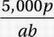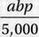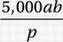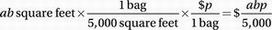# SAT Math Multiple Choice Question 623: Answer and Explanation

### Test Information

Question: 623

8. One bag of grass seed can cover 5,000 square feet of new lawn. If each bag costs p dollars, which of the following expressions gives the cost, in dollars, to cover a new rectangular lawn that measures a feet by b feet?

• A.• B.• C.• D. 5,000abp

Explanation:

B

Algebra (word problems) MEDIUM

Perhaps the most straightforward way to approach this question is to regard it as a conversion from a given area of lawn (in square feet) to cost (in dollars).

Area of rectangular lawn:

A = bh = ab square feet

Convert using given conversion factors:Make sure to check this calculation by noticing that all units "cancel" as common factors, except for the unit we want, dollars, which remains in the numerator.